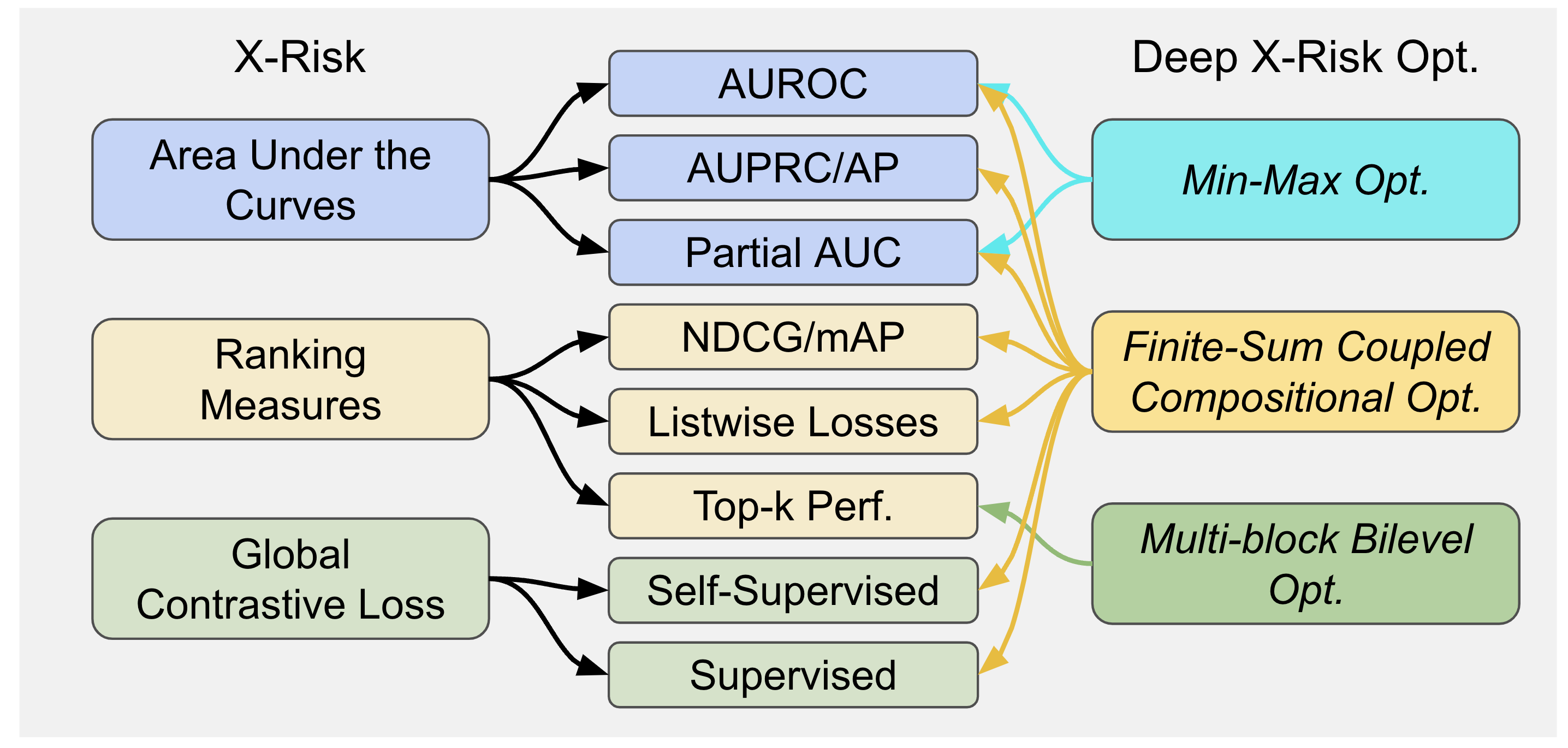# A Deep Learning Library for X-Risk Optimization

## Why LibAUC?

LibAUC is a novel deep learning library to offer an easier way to directly optimize commonly used performance measures and losses with user-friendly APIs. LibAUC has broad applications in AI for tackling both classic and emerging challenges, such as Classification of Imbalanced Data (CID), Learning to Rank (LTR), and Contrastive Learning of Representation (CLR).

LibAUC provides a unified framework to abstract the optimization of a family of risk functions called X-Risk, including surrogate losses for AUROC, AUPRC/AP, and partial AUROC that are suitable for CID, surrogate losses for NDCG, top-K NDCG, and listwise losses that are used in LTR, and global contrastive losses for CLR. Here’s an overview:For more details, please check our LibAUC paper.

## What is X-Risk?

LibAUC is powered by Deep X-Risk Optimization (DXO), where X-Risk formally refers to a family of compositional measures in which the loss function of each data point is defined in a way that contrasts the data point with a large number of others. Mathematically, X-Risk optimization can be cast into the following abstract optimization problem:

$\min _{\mathbf{w} \in \mathbb{R}^d} F(\mathbf{w})=\frac{1}{|\mathcal{S}|} \sum_{\mathbf{z}_i \in \mathcal{S}} f_i\left(g\left(\mathbf{w} ; \mathbf{z}_i, \mathcal{S}_i\right)\right)$

where $$g: \mathbb{R}^d \mapsto \mathcal{R}$$ is a mapping, $$f_i: \mathcal{R} \mapsto \mathbb{R}$$ is a simple deterministic function, $$\mathcal{S}=\left\{\mathbf{z}_1, \ldots, \mathbf{z}_m\right\}$$ denotes a target set of data points, and $$\mathcal{S}_i$$ denotes a reference set of data points dependent or independent of $$\mathbf{z}_i$$. For mathmetrical derviations, please check the DXO paper.

Get Started

## Reference

If any questions, please reach out to Zhuoning Yuan and Tianbao Yang. If you find our works helpful, please consider citing the following papers:

@inproceedings{yuan2023libauc,
title={LibAUC: A Deep Learning Library for X-risk Optimization.},
author={Zhuoning Yuan and Dixian Zhu and Zi-Hao Qiu and Gang Li and Xuanhui Wang and Tianbao Yang},
booktitle={29th SIGKDD Conference on Knowledge Discovery and Data Mining},
year={2023}}

@article{yang2022algorithmic,
title={Algorithmic Foundation of Deep X-risk Optimization},
author={Yang, Tianbao},
journal={arXiv preprint arXiv:2206.00439},
year={2022}}